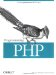# 5.10 Using Arrays

Arrays crop up in almost every PHP program. In addition to their obvious use for storing collections of values, they're also used to implement various abstract data types. In this section, we show how to use arrays to implement sets and stacks.

#### 5.10.1 Sets

Arrays let you implement the basic operations of set theory: union, intersection, and difference. Each set is represented by an array, and various PHP functions implement the set operations. The values in the set are the values in the array the keys are not used, but they are generally preserved by the operations.

The union of two sets is all the elements from both sets, with duplicates removed. The array_merge( ) and array_unique( ) functions let you calculate the union. Here's how to find the union of two arrays:

`function array_union(\$a, \$b) {   \$union = array_merge(\$a, \$b); // duplicates may still exist   \$union = array_unique(\$union);   return \$union; } \$first = array(1, 'two', 3); \$second = array('two', 'three', 'four'); \$union = array_union(\$first, \$second); print_r(\$union); Array (      => 1      => two      => 3      => three      => four )`

The intersection of two sets is the set of elements they have in common. PHP's built-in array_intersect( ) function takes any number of arrays as arguments and returns an array of those values that exist in each. If multiple keys have the same value, the first key with that value is preserved.

Another common function to perform on a set of arrays is to get the difference; that is, the values in one array that are not present in another array. The array_diff( ) function calculates this, returning an array with values from the first array that are not present in the second.

The following code takes the difference of two arrays:

`\$first = array(1, 'two', 3); \$second = array('two', 'three', 'four'); \$difference = array_diff(\$first, \$second); print_r(\$difference); Array (      => 1      => 3 )`

#### 5.10.2 Stacks

Although not as common in PHP programs as in other programs, one fairly common data type is the last-in first-out (LIFO) stack. We can create stacks using a pair of PHP functions, array_push( ) and array_pop( ). The array_push( ) function is identical to an assignment to \$array[]. We use array_push( ) because it accentuates the fact that we're working with stacks, and the parallelism with array_pop() makes our code easier to read. There are also array_shift( ) and array_unshift( ) functions for treating an array like a queue.

Stacks are particularly useful for maintaining state. Example 5-4 provides a simple state debugger that allows you to print out a list of which functions have been called up to this point (i.e., the stack trace).

##### Example 5-4. State debugger
`\$call_trace = array(  ); function enter_function(\$name) {   global \$call_trace;   array_push(\$call_trace, \$name); // same as \$call_trace[] = \$name   echo "Entering \$name (stack is now: " . join(' -> ', \$call_trace) . ')<br />'; } function exit_function(  ) {   echo 'Exiting<br />';   global \$call_trace;   array_pop(\$call_trace);        // we ignore array_pop(  )'s return value } function first(  ) {   enter_function('first');   exit_function(  ); } function second(  ) {   enter_function('second');     first(  );   exit_function(  ); } function third(  ) {   enter_function('third');     second(  );     first(  );   exit_function(  ); } first(  ); third(  );`

Here's the output from Example 5-4:

` Entering first (stack is now: first) Exiting Entering third (stack is now: third) Entering second (stack is now: third -> second) Entering first (stack is now: third -> second -> first) Exiting Exiting Entering first (stack is now: third -> first) Exiting Exiting Exiting`Programming PHP
ISBN: 1565926102
EAN: 2147483647
Year: 2007
Pages: 168

Similar book on Amazon